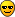# Problems with Erf function

Dear all.
I am trying to fit a plot with a function.
The fitting function is the convolution of a 2D Gaussian and a step function in 2D and is reported in the attachment.

The implementation of the fitting function is the following:

Double_t GausErf(Double_t x, Double_t par)
{
//if(par > 0 && par > 0)
if((TMath::Sqrt((x-par)
(x-par)+(x-par)
(x-par)))<1.// Rmax = 1.8 cm
{
double rx=0.;
double ry=0.;
double rz=0.;
double den=0;
double g=0;
rx=(x-par)/par;
ry=(x-par)/par;
rz=2.parrxry;
den= 1.-parpar;
g=TMath::Sqrt( (rx
rx +ry
ry - rz)/(2.*den) );
double fitval = (par/ (2.*par)) * (TMath::Erf(par+g) - TMath::Erf(g-par));
return fitval;
}
else
return 0;
}

Is there something wrong in this implementation, please?
When I fit in the following way

TH2D h_image = (TH2D)f_image->Get(histoname);
TF2 *g2D=new TF2(“g2d”,GausErf,h_image->GetMean(1)-3,h_image->GetMean(1)+3,h_image->GetMean(2)-3,h_image->GetMean(2)+3,7);
g2D->SetParNames(“Const”,“X_{0}”,"#sigma_{x}",“Y_{0}”,"#sigma_{y}","#rho",“a”);
g2D->SetParameters(0,0,0.5,0,0.5,0.,0);
h_image->Fit(g2D,“R0+”);

I obtain

TF2 *g2D=new TF2(“g2d”,GausErf,h_image->GetMean(1)-lim,h_image->GetMean(1)+lim,h_image->GetMean(2)-lim,h_image->GetMean(2)+lim,7);
g2D->SetParNames(“Const”,“X_{0}”,"#sigma_{x}",“Y_{0}”,"#sigma_{y}","#rho",“a”);
g2D->SetParameters(0,0,0.5,0,0.5,0.,0); // 2010 data
h_image->Fit(g2D,“R0+”);
cout << TMath::Erf(2)<<endl;

I am using root 5.32/01 and the function I am trying to fit with Gaus2DErf is a 2D Gaussian.
Since I will have to fit another function with Gaus2DErf, I am testing the fit on the 2D Gaussian, expectin the term “a” to be zero, but the fit does not work.